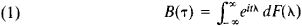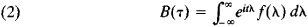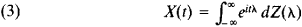# Stationary Stochastic Process

Also found in: Dictionary, Thesaurus, Wikipedia.
Related to Stationary Stochastic Process: nonstationary

## stationary stochastic process

[′stā·shə‚ner·ē stō′kas·tik ′prä·səs]
(mathematics)
A stochastic process x (t) is stationary if each of the joint probability distributions is unaffected by a change in the time parameter t.
McGraw-Hill Dictionary of Scientific & Technical Terms, 6E, Copyright © 2003 by The McGraw-Hill Companies, Inc.
The following article is from The Great Soviet Encyclopedia (1979). It might be outdated or ideologically biased.

## Stationary Stochastic Process

an important special class of stochastic processes that is often encountered in applications of probability theory in various branches of science and engineering. A stochastic process X(t) is said to be stationary if the probabilistic quantities characterizing the process are independent of time t. For example, the probability distribution of X(t) is the same for all t, and the joint probability distribution of X(t1) and X(t2) depends only on the length of the time interval t2t1 — that is, the distributions of the pairs of variables {X(t1), X(t2)} and {X(t1 + s), X(t2 + s)} are identical for any (t1, t2, and s.

Stationary stochastic processes are good approximations of many actual phenomena involving irregular fluctuations. For example, electric noise, or fluctuations of current or voltage, in an electric circuit can be regarded as a stationary stochastic process if the circuit is in a steady-state condition—that is, if all the circuit’s macroscopic characteristics and all the conditions causing the flow of current do not change with time. Similarly, the velocity fluctuations at a point in a turbulent flow are a stochastic process if the general conditions producing the flow do not vary—that is, if the flow is a steady one. These and other cases of stationary stochastic processes in physics (particularly geophysics and astrophysics), mechanics, and engineering stimulated the development of research on such processes. Important generalizations of the concept of a stationary stochastic process include the stochastic process with stationary increments of a given order, the generalized stationary stochastic process, and the homogeneous random field.

The moments of the probability distributions of the values of the process X(t) play a fundamental role in the mathematical theory of stationary stochastic processes. These moments are the simplest numerical quantities characterizing the distributions. The first- and second-order moments are especially important. The first-order moment, which is the mathematical expectation of the random variable X(t), is the mean value of the stationary stochastic process: EX(t) = m. The second-order moment, which is the mathematical expectation of the product X(t1) X(t2), is the correlation function of the stationary stochastic process: EX(t1)X(t2) = B(t2 ’ t1). This expectation can be expressed simply in terms of the variance of the variables X(t) and the correlation coefficient of X(t1) and X(t2) (seeCORRELATION). Many mathematical investigations of stationary stochastic processes concentrate on those properties of the process that are completely determined by just m B(τ). In this connection the stochastic process X(t) is often said to be stationary in the wide sense if the mean value EX(t) = m is constant and the correlation function B(t2, t1) = EX(t1)X(t2) depends only on t2t1 If all the characteristics of the stochastic process are independent of time, the process is then said to be strictly stationary, or stationary in the strict sense.

Studies based on the representation of the stochastic process X(t) and its correlation function B(t2 ’ tx) = B(τ) as a Fourier or Fourier-Stieltjes integral are of great importance in the mathematical theory of stationary stochastic processes. A fundamental role is played here by the Khinchin theorem: the correlation function of the stationary stochastic process X(t) can always be represented in the formwhere F(λ) is a monotonic nondecreasing function of λ and the integral on the right is a Stieltjes integral. If B(τ) decreases rapidly enough as ǀτǀ → ∞ [as is most often the case in applications when X(t) is understood as the difference X(t)−m], then the integral on the right side of (1) becomes an ordinary Fourier integral:where f(λ) = F’(λ) is a nonnegative function. The function F(λ) is called spectral function of the stationary stochastic process X(t), and f(λ), when (2) holds, is called the spectral density of the process. It also follows from the Khinchin theorem that the process X(t) itself admits of a spectral representation of the formwhere Z(λ) is a random function with uncorrelated increments and the integral on the right is understood as the mean square limit of the corresponding sequence of integral sums. The representation (3) permits us to regard any stationary stochastic process X(t) as the superposition of uncorrelated harmonic oscillations of distinct frequencies with random amplitudes and phases. Here, the spectral function F(λ) and spectral density f(λ) determine the distribution of the mean energy of the harmonic oscillations in A”(i) with respect to the spectrum of frequencies X. In applied research, f(λ) is often called the power spectrum of the stationary stochastic process X(t).

E. E. Slutskii introduced the concept of the stationary stochastic process and obtained the first mathematical results concerning such processes in the late 1920’s and early 1930’s. Important studies on the theory of stationary stochastic processes were subsequently made by such mathematicians as A. Ia. Khinchin, A. N. Kolmogorov, H. Cramer, and N. Wiener.

### REFERENCES

Slutskii, E. E. Izbr. tr. Moscow, 1960.
Khinchin, A. Ia. “Teoriia korreliatsii statsionarnykh stokhasticheskikh protsessov.” Uspekhi matematicheskikh nauk, 1938, fasc. 5, pp. 42–51.
Rozanov, Iu. A. Statsionarnye sluchainyeprotsessy. Moscow, 1963.
Prokhorov, Iu. V., and Iu. A. Rozanov. Teoriia veroiatnostei (Osnovnye poniatiia, Predel’nye teoremy, Sluchainye protsessy), 2nd ed. Moscow, 1973.
Gikhman, I. I., and A. V. Skorokhod. Teoriia sluchainykh protsessov, vol. 1. Moscow, 1971.
Hannan, E. Mnogomernye vremennye riady. Moscow, 1974. (Translated from English.)

A. M. IAGLOM